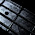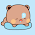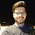Saturday, May 22, 2010

Maximum bipartite matching in a bipartite graph

Although Hopcroft Karp is faster and smarter, this one is pretty simple to code specially in contest time and when the graph is relatively smaller. It uses a DFS subroutine to cut and establish matching and thus produces a maximum matching. This version of BPM takes the adjacency list of left side of the bipartite graph and updates the Left[] and Right[] arrays with their respective matches.

Here, in the DFS subroutine, there are two for loops, where, the first one checks for yet unestablished connections, and the second one is the recursive DFS step. These two steps could be written in a single loop and the condition modified as "( Right[v]==-1 || dfs(Right[v]) )", actually separating them increases performance by some factors, because, first time it checks all the unmatched nodes before going into DFS.

A sample C++ implementation:
`#define SET(x) memset(x, -1, sizeof(x))#define CLR(x) memset(x, 0, sizeof(x))#define MAX 100vector < int > edges[MAX];bool visited[MAX];int Left[MAX], Right[MAX];bool dfs(int u) {    if(visited[u]) return false;    visited[u] = true;    int len = edges[u].size(), i, v;    for(i=0; i<len; i++) {        v = edges[u][i];        if(Right[v]==-1) {            Right[v] = u, Left[u] = v;            return true;        }    }    for(i=0; i<len; i++) {        v = edges[u][i];        if(dfs(Right[v])) {            Right[v] = u, Left[u] = v;            return true;        }    }    return false;}int match() {    SET(Left);    SET(Right);    int i, ret = 0;    bool done;    do {        done = true;        CLR(visited);        for(i=0; i<MAX; i++) {            if(Left[i]==-1 && dfs(i)) {                done = false;            }        }    } while(!done);    for(i=0; i<MAX; i++) ret += (Left[i]!=-1);    return ret;}`

Notes: Here, edges[MAX] is the left side adjacency list, implemented with vector, Left[MAX] and Right[MAX] holds the matching and the procedure match() returns maximum matching. Pretty straight forward.

1.Hello.

Can you describe, how this code works? I don´t understand what edges really is. Will this code compute the maximum number of possible pairings?

1.edges is an array of vectors (C++), it is used to hold the adjacency list representation here. So, when you are constructing the graph, if you have an edge u -> v, you have to code like:
edges[u].push_back(v); // adds an edge u->v
edges[v].push_back(u); // needed only if the graph edges are bi-directional

and yes, it returns the maximal possible pairing.

2.Sir, I couldn't understand your representation of holding the matching in arrays LEFT[] and RIGHT[]. It would be great help if you could elaborate little more on what does LEFT[ i ] and RIGHT[ j ] signify?

1.Left[i] holds the index of the right side node to which it is matched. Vice versa for Right[j].

3.OK. I got it.. Thanx :)